##### Quantum Physics For Dummies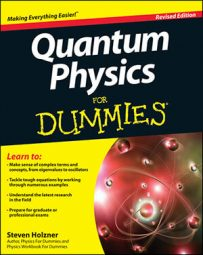In quantum physics, if you’re given an operator in matrix form, you can find its eigenvectors and eigenvalues. For example, say you need to solve the following equation: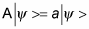First, you can rewrite this equation as the following: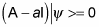I represents the identity matrix, with 1s along its diagonal and 0s otherwise: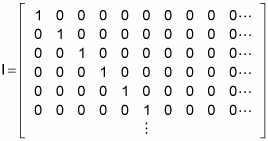Remember that the solution to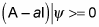exists only if the determinant of the matrix A – aI is 0:

det(A – aI) = 0

## How to find the eigenvalues

Any values of a that satisfy the equation det(A – aI) = 0 are eigenvalues of the original equation. Try to find the eigenvalues and eigenvectors of the following matrix: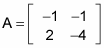First, convert the matrix into the form A – aI: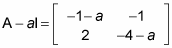Next, find the determinant: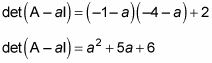And this can be factored as follows: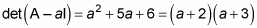You know that det(A – aI) = 0, so the eigenvalues of A are the roots of this equation; namely, a1 = –2 and a2 = –3.

## How to find the eigenvectors

How about finding the eigenvectors? To find the eigenvector corresponding to a1, substitute a1 — the first eigenvalue, –2 — into the matrix in the form A – aI: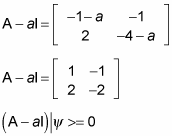So you have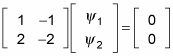Because every row of this matrix equation must be true, you know that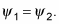And that means that, up to an arbitrary constant, the eigenvector corresponding to a1 is the following: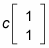Drop the arbitrary constant, and just write this as a matrix: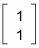How about the eigenvector corresponding to a2? Plugging a2, –3, into the matrix in A –aI form, you get the following: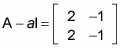Then you have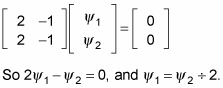And that means that, up to an arbitrary constant, the eigenvector corresponding to a2 is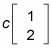Drop the arbitrary constant: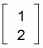So the eigenvalues of this matrix operatorare a1 = –2 and a2 = –3. And the eigenvector corresponding to a1 isThe eigenvector corresponding to a2 is